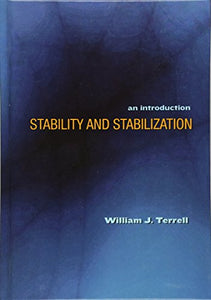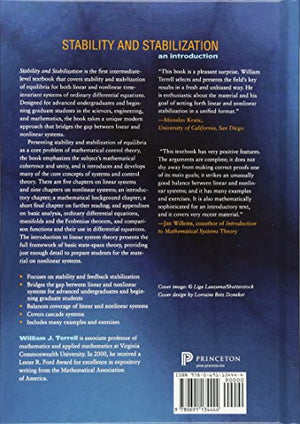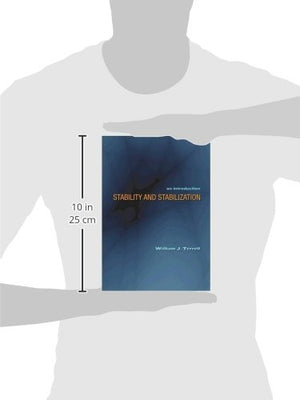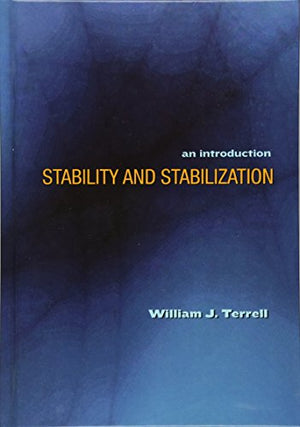# Stability And Stabilization: An Introduction

• Publish Date: 2009-02-15
• Binding: Hardcover
Regular price \$69.97

Attention: For textbook, access codes and supplements are not guaranteed with used items.

Stability and Stabilization is the first intermediate-level textbook that covers stability and stabilization of equilibria for both linear and nonlinear time-invariant systems of ordinary differential equations. Designed for advanced undergraduates and beginning graduate students in the sciences, engineering, and mathematics, the book takes a unique modern approach that bridges the gap between linear and nonlinear systems.

Presenting stability and stabilization of equilibria as a core problem of mathematical control theory, the book emphasizes the subject's mathematical coherence and unity, and it introduces and develops many of the core concepts of systems and control theory. There are five chapters on linear systems and nine chapters on nonlinear systems; an introductory chapter; a mathematical background chapter; a short final chapter on further reading; and appendixes on basic analysis, ordinary differential equations, manifolds and the Frobenius theorem, and comparison functions and their use in differential equations. The introduction to linear system theory presents the full framework of basic state-space theory, providing just enough detail to prepare students for the material on nonlinear systems.

• Focuses on stability and feedback stabilization